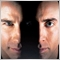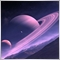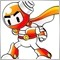# 请问根据sar信号平仓怎么写？51

MT4平台，求大神帮帮忙43

Dongmin Zhou:

MT4平台，求大神帮帮忙

SAR空头和多头，你是怎么定义的？K线收盘价大于SAR，就是多头，还是有什么其它的说法？详细描述，才好办，加我好友吧51

1111.png 7 kb264651

Shaofei Chen:

if(iSAR(OrderSymbol(),x,S1,S2,0)>=iHigh(OrderSymbol(),Cyc,0) && iSAR(OrderSymbol(),x,S1,S2,1)<=iLow(OrderSymbol(),x,1))

if(iSAR(OrderSymbol(),x,S1,S2,0)<=iLow(OrderSymbol(),x,0) && iSAR(OrderSymbol(),x,S1,S2,1)>=iHigh(OrderSymbol(),x,1))6

Dongmin Zhou:

if(iSAR(OrderSymbol(),x,S1,S2,0)>=iHigh(OrderSymbol(),Cyc,0) && iSAR(OrderSymbol(),x,S1,S2,1)<=iLow(OrderSymbol(),x,1))

if(iSAR(OrderSymbol(),x,S1,S2,0)<=iLow(OrderSymbol(),x,0) && iSAR(OrderSymbol(),x,S1,S2,1)>=iHigh(OrderSymbol(),x,1))651

bingshanyijiao:1337